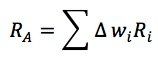### Why should I choose AnalystNotes?

AnalystNotes specializes in helping candidates pass. Period.

##### Subject 3. The Fundamental Law of Active Management
The fundamental law is a framework to evaluate the potential value added by various investment strategies.

Active Security Returns

Most portfolio managers appreciate the fact that value added ultimately depends on their ability to correctly forecast security returns.

The correlation triangle characterizes the relationships between forecasted and realized returns and active security weights.The base represents the value added through active management. Value added is measured by the active return:This indicates that value is added when positive-active-weight securities have positive residual returns and negative-active-weight securities have negative residual returns. In other words, performance in any given period is related to the cross-sectional correlation between the active security weights and realized residual returns - the security data in the bottom two corners of the correlation triangle.

There is little hope of value added if the manager's forecasts of returns do not correspond to actual realized returns. Signal quality is measured by the relationship between the forecasted active returns at the top of the triangle and the realized active returns at the right corner. The cross-sectional correlation is commonly called the information coefficient (IC).

• IC can take on values anywhere from -1 to 1.
• The ex ante IC must be positive, or the investor would generally not pursue active management.

Managers with a high information coefficient (ability to forecast returns) will add more value over time - but only to the extent that those forecasts are exploited in the construction of the managed portfolio. The second correlation, the relationship between the active weights in the left corner and forecasted active returns at the top of the triangle, measures the degree to which managers' forecasts are translated into active weights. The cross-sectional correlation is referred to as the transfer coefficient (TC).

The Basic Fundamental Law

The law describes an ex ante relationship between expected performance and the assumed IC of the manager's forecasting process.where BR is the breadth, or number of independent "bets" in the actively managed portfolio. It is the number of times per year a manager can use his/her skill. The breadth measures diversification. The information coefficient measures the quality of these decisions.

Another form of the equation is:The important lesson of the fundamental law is that breadth of application, as well as the quality of the signal, dictates the value to be added through active management.

The Full Fundamental Law

TC is equal to 1 in the absence of constraints in portfolio construction. However, portfolio constraints such as no short sales and mandated industry or sector concentrations limit the full transfer of information into active weights and lead to TC lower than 1.

Including the impact of the transfer coefficient, the full fundamental law becomes:Like the information coefficient, the transfer coefficient acts as a simple scaling factor in the determination of value added. One can think of the transfer coefficient as an additional adjustment to breadth that reflects the reduction in independent bets because of constraints.

The full fundamental law is intuitive. The expected active return is the product of four key parameters:

• The transfer coefficient, which measures the structuring of the portfolio
• The information coefficient, which measures the manager's skill
• Breadth, which measures the number of independent, active decisions available to an active manager per year
• Portfolio active risk, which measures the level of aggressiveness

Learning Outcome Statements

describe and interpret the fundamental law of active portfolio management including its component terms - transfer coefficient, information coefficient, breadth, and active risk (aggressiveness);

CFA® 2023 Level II Curriculum, Volume 6, Module 45